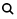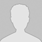#学习小专题

## 离子浓度大小比较题型归类解析

A．c(Na)＝2c(CO32) 　　　　　　　　B．c(OH)＝2c(H)

C．c(HCO3)＞c(H2CO3) 　　　　　　　 D．c(Na)＜c(CO32)＋c(HCO3)

A．c(K)＞c(Al3＋)

B．c(K)＝c(Al3＋)

C．c(H)＝c(OH)

D．c(Al3＋)＜c(H)

A．c(Cl)＞c(NH4)＞c(H)＞c(OH)

B．c(NH4)＞c(Cl)＞c(H)＞c(OH)

C．c(Cl)＝c(NH4)＞c(H)＝c(OH)

D．c(NH4)＝c(Cl)＞c(H)＞c(OH)

1、恰好中和型

A．c(Na)＞c(Ac)＞c(H)＞c(OH)

B．c(Na)＞c(Ac)＞c(OH)＞c(H)

C．c(Na)＝c(Ac)＋c(HAc)

D．c(Na)＋c(H)＝c(Ac)＋c(OH)

A．c(M)＞c(OH)＞c(A)＞c(H)

B．c(M)＞c(A)＞c(H)＞c(OH)

C．c(M)＞c(A)＞c(OH)＞c(H)

D．c(M)＋c(H)＝c(A)＋c(OH)

2、pH等于7型

(1)考虑从电荷守恒入手求得其它离子相等关系。

(2)判断pH等于7时，酸碱到底谁过量；方法是：先设定为完全反应时的pH然后与pH=7比较便可得出。

A．c(HCOO)＞c(Na)

B．c(HCOO)＜c(Na)

C．c(HCOO)＝c(Na)

D．无法确定c(HCOO)与c(Na)的关系

A．c(A)＝c(K)

B．c(H)＝c(OH)＜c(K)＜c(A)

C．Ｖ≥20mL

D．Ｖ≤20mL

(1)假设两考等体积混合则，则电离出来的n(H)和n(OH)恰好反应完全但此时及有酸(pH=4时，酸的浓度大于1×10－4mol/L)pH＜7，故为了使pH=7必须少加酸，故Ｖ≤20mL

(2)因c(HA)＞10－4mol・L－1，故加入Ｖ(HA)＜１0mL 即达pH=7，此时Ｖ≤20mL，综合上述，Ｖ≤20mL，D项也正确。

【变式训练】在常温下10mL 0.01mol/L 的KOH溶液中，加入0.01mol/L 的一元酸HA溶液至pH刚好等于7(假设反应前后体积不变)，则对反应后溶液的叙述正确的是( )。

A．c(A)＝c(K)

B．c(H)＝c(OH)＜c(K)＜c(A)

C．Ｖ≥20mL

D．Ｖ≤20mL

3、反应过量型

A．c(HCN)＜c(CN)

B．c(Na)＞c(CN)

C．c(HCN)－c(CN)＝c(OH)

D．c(HCN)＋c(CN)＝0.1mol・L－1

A．c(CH3COO)＞c(Na)

B．c(CH3COOH)＞c(CH3COO)

C．2c(H)＝c(CH3COO)－c(CH3COOH)

D．c(CH3COOH)＋c(CH3COO)＝0.01mol・L－1

A．c(NH4)＞c(Cl)＞c(H)＞c(OH)

B．c(NH4)＞c(Cl)＞c(OH)＞c(H)

C．c(Cl)＞c(NH4)＞c(H)＞c(OH)

D．c(Cl)＞c(NH4)＞c(OH)＞c(H)

A．c(CH3COO)＞c(Cl)＞c(H)＞c(CH3COOH)

B．c(CH3COO)＞c(Cl)＞c(CH3COOH)＞c(H)

C．c(CH3COO)＝c(Cl)＞c(H)＞c(CH3COOH)

D．c(Na)＋c(H)＝c(CH3COO)＋c(Cl)＋c(OH)

A．c(Na)＞c(Cl)＞c(OH)＞c(H)

B．c(Na)＝c(Cl)＞c(OH)＞c(H)

C．c(Na)＝c(Cl)＞c(H)＞c(OH)

D．c(Cl)＞c(Na)＞c(OH)＞c(H)

A．NH4Cl　　　　　B．NH4HSO4　　　C．CH3COONH4　　　　　D．NH4HCO3

1．下列混合溶液中，各离子浓度的大小顺序正确的是()

A．10mL0・1mol•L－1氨水与10mL0・1mol•L－1盐酸混合，c(Cl)＞c(NH4)＞c(OH)＞c(H)，

B．10mL0・1mol•L－1NH4Cl溶液与5mL0・2mol•L－1NaOH溶液混合，c(Na)=c(Cl)＞c(OH)＞c(H)

C．10mL0・1mol•L－1CH3COOH溶液与5mL0・2mol•L－1NaOH溶液混合，c(Na)=c(CH3COO)＞c(OH)＞c(H)

D．10mL0・5mol•L－1NaOH溶液与6mL1mol•L－1盐酸混合，c(Cl)＞c(Na)＞c(OH)＞c(H)

2．将0.2mol・L－1HCN溶液和0.1mol・L－1的NaOH溶液等体积混合后，溶液显碱性，下列关系式中正确的是(   )

A.c(HCN)＜c(CN)

B.c(Na)＞c(CN)

C.c(HCN)－c(CN)＝c(OH)

D.c(HCN)＋c(CN)＝0.1mol・L－1

3．将20mL0・4mol・L－1硝酸铵溶液跟50mL0・1mol・L－1氢氧化钡溶液混合，则混合溶液中各离子浓度的大小顺序是()

A．c(NO3)＞c(OH)＞c(NH4)＞c(Ba2＋)

B．c(NO3)＞ c(Ba2＋)＞ c(OH)＞c(NH4)

C．c(Ba2＋) ＞ c(NO3-)＞c(OH)＞c(NH4)

D．c(NO3)＞ c(Ba2＋)＞c(NH4)＞ c(OH)

4．在10mL 0.1mol・L－1NaOH溶液中加入同体积、同浓度的HAc溶液，反应后溶液中各微粒的浓度关系错误的是()

A．c(Na)＞c(Ac)＞c(H)＞c(OH)

B．c(Na)＞c(Ac)＞c(OH)＞c(H)

C．c(Na)=c(Ac)＋c(HAc)

D．c(Na)＋c(H)=c(Ac)＋c(OH)

5．常温下，把pH＝11的NaOH溶液与pH＝3的醋酸溶液等体积混合，在所得溶液中离子浓度大小关系正确的是()

A．c(CH3COO)＞c(Na)＞c(H)＞c(OH)

B．c(Na)＋c(H)＞c(CH3COO)＋c(OH)

C．c(CH3COO)＞c(Na)＞c(H)＝c(OH)

D．c(Na)＞c(CH3COO)＞c(OH)＞c(H)

6．常温下，将甲酸和氢氧化钠溶液混合，所得溶液 pH＝7，则此溶液中 ()

Ac(HCOO)＞c(Na)

Bc(HCOO)＜c(Na)

Cc(HCOO)＝c(Na)

D无法确定c(HCOO)与C(Na)的关系

7．将0.1mol/L 的醋酸钠溶液20mL 与0.1mol/L 盐酸10HCl混合后，溶液显酸性，则溶液中有关微粒的浓度关系正确的是()

A.c (Ac)＞c (Cl)＞c (H)＞c (HAc)

B.c (Ac)＞c (Cl)＞c (HAc)＞c(H)

C.c (Ac)＝c (Cl)＞c (H)＞c (HAc)

D.c (Na)＋c (H)＝c (Ac)＋c (Cl)＋c (OH)

8．在甲酸溶液中加入一定量NaOH溶液，恰好完全反应，对于生成的溶液，下列判断一定正确的是()

A．c(HCOO)＜c(Na)

B．c(HCOO)＞c(Na)

C．c(OH)＞c(HCOO)

D．c(OH)＜c(HCOO)

9．把0.02mol/L HAc溶液和0.01mol/L NaOH溶液以等体积混和，则混合液中微粒浓度关系正确的为()

(A) c(Ac)＞c(Na)

(B) c(HAc)＞c(Ac)

(C)2c(H)=c(Ac)－c(HAc)

(D)c(HAc)＋c(Ac)=0.01mol/L

10．将0.1mol・L－1CH3COOH溶液加水稀释或加入少量CH3COONa晶体时，都会引起( )

A溶液的pH增加

BCH3COOH电离程度变大

C溶液的导电能力减弱

D溶液中c(OH)减少

11．将标准状况下的2．24L CO2通入150mL1mol/LNaOH溶液中，下列说法正确的是( )

A．c(HCO3)略大于c(CO32)

B．c(HCO3)等于c(CO32)

C．c(Na)等于c(CO32)与c(HCO3)之和

D．c(HCO3)略小于c(CO32)

12．在 0.1 mol/L Na2CO3溶液中，下列关系正确的是( )

A．c(Na) = 2c(CO32)

B．c(OH) = 2c(H)

C．c(HCO3) ＞ c(H2CO3)

D．c(Na) ＜〔c(CO32)＋c(HCO3) 〕

13．草酸是二元弱酸，草酸氢钾溶液呈酸性。在0.1mol・L－1KHC2O4溶液中，下列关系正确的( )

A．c(K)＋c(H)=c(HC2O4)＋c(OH)＋c(C2O42)

B．c(HC2O4)＋c(C2O42)=0.1 mol・L－1

C．c(C2O42)＞c(H2C2O4)

D．c(K)=c(H2C2O4)＋c(HC2O4)＋c(C2O42)

14．已知0.1mol・L－1的二元酸H2A溶液的pH=4.0，则下列说法中正确的是()

A．在Na2A、NaHA两溶液中，离子种类不相同

B．在溶质物质的量相等的Na2A、NaHA两溶液中，阴离子总数相等

C．在NaHA溶液中一定有：c(Na)＋c(H+)=c(HA)＋c(OH)＋2c(A2-)

D．在Na2A溶液中一定有：c(Na)＞C(A2－)＞ c(H)＞c(OH)

15．甲酸和乙酸都是弱酸，当它们的浓度均为0.10mol・L－1时，甲酸中的c(H)约为乙酸中C(H)的3倍。现有两种浓度不等的甲酸溶液A和B，以及0.10mol・L－1的乙酸，经测定它们的pH从大到小依次为A、乙酸、B。由此可知()

A．A的浓度必小于乙酸的浓度

B．A的浓度必大于乙酸的浓度

C．B的浓度必小于乙酸的浓度

D．B的浓度必大于乙酸的浓度

16．下列说法正确的是()

A．pH=2和pH=1的硝酸中c(H)之比为1：10

B．Na2CO3溶液c(Na)与c(CO32)之比为2：1

C．0.2 mol・L－1与0.1 mol・L－1醋酸中c(H)之比为2：1

D．NO2溶于水时，被氧化的n(NO2)与被还原的n(NO2)之比为3：1

17．将0.L mol・ 醋酸溶液加水稀释，下列说法正确的是()

A．溶液中c(H)和c(OH)都减小

B．溶液中c(H)增大

C．醋酸电离平衡向左移动

D．溶液的pH增大

18．在常温下用水稀释0.1 mol・L－1的醋酸钠溶液，溶液中增大的是()

A.c(H)

B.c(H)・c(OH

C. c(OH)

D. c(H)/c(CH3COOH)

19．常温下实验测得 0.Lmol／L某一元酸 HA溶液的 pH值≠1，0.Lmol／L某一元碱 BOH溶液里 C( H)／C( OH)＝10－12.将此两种溶液等体积混合后所得溶液中各离子浓度间关系正确的是 ( )

A．c(A)＞c(B)＞c(H)＞c( OH)

B．c(B)＞c(A)＞c( OH)＞c(H)

C．c(A)=c(B)＞c(H)=c( OH)

D．c(B)＋ c(H)=c(A)＋c( OH)

20．下列各组数据关系中，前者比后者大的是( )

A．纯水100℃和25℃时的pH

B．Na2CO3溶液中的HCO3-和OH-数目

C．常温下，pH= 1的 KOH溶液和 pH= 11的CH3COK溶液中由水电离出的OH一离子浓度

D．100mL0.Lmol/L的CH3COOH溶液与10mL0.Lmol/L的CH3COOH溶液的H数目

 1 2 3 4 5 6 7 8 9 10 B BD B A A C BD AD AD A 11 12 13 14 15 16 17 18 19 20 A C Cd C A A D AD BD D

(责任编辑：)

------分隔线----------------------------

------分隔线----------------------------

 离子浓度大小比较之三大守恒专题 离子浓度大小比较之分布曲线专练 离子浓度大小比较之水的电离曲线 离子浓度大小比较之三大守恒专题 离子浓度大小比较之强碱滴定二元 离子浓度大小比较之对数曲线专练 用好“四点”突破图像类溶液中微 微课视频之乐乐课堂：盐类水解反 常见盐溶液中离子浓度的精准算法 2008～2017江苏高考离子浓度比较 离子浓度的大小比较专题文档 微课视频：溶液中粒子浓度大小比 微课视频：离子浓度大小比较 电解质溶液中微粒浓度大小比较的 溶液中离子浓度大小比较的常见错 溶液中离子浓度大小的比较及其规 电解质溶液稀释时离子浓度的变化 离子浓度大小比较一、二、三、四• 全部评论（0
还没有评论，快来抢沙发吧！